# How to convert numbers to text in excel

Feb 04, 2020 • edited Feb 05, 2020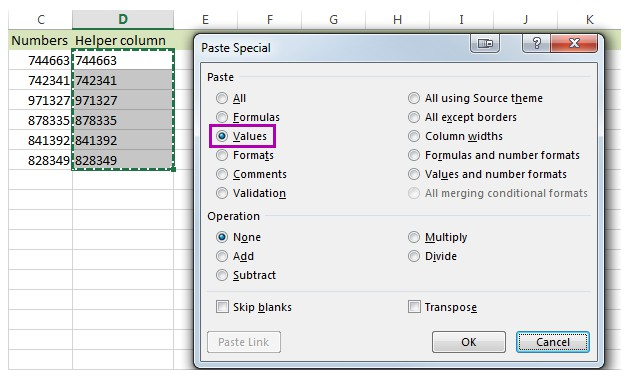If you use Excel spreadsheets to store long and not so long numbers, one day you may need to convert them to text. There may be different reasons to change digits stored as numbers to text.

Sometimes you may not want the numbers in the cells getting involved in calculating, or you may want to display leading zeros in numbers in cells. For doing so, you may need to change a number into text.

# Numbers to Text

By default, numbers are right-aligned and text is left-aligned. This example teaches you how to convert numbers to ‘text strings that represent numbers’.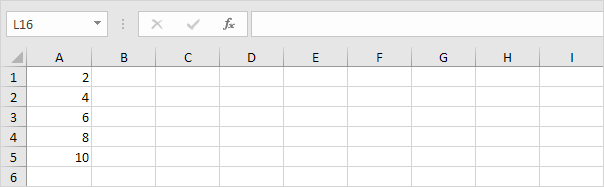1. Select the range A1:A4 and change the number format to Text.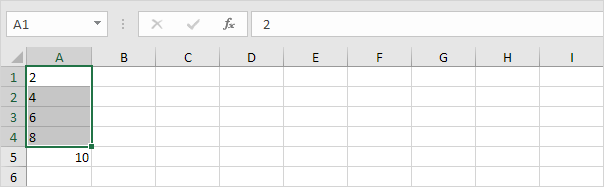2. Precede a number by an apostrophe and it will also be treated as text.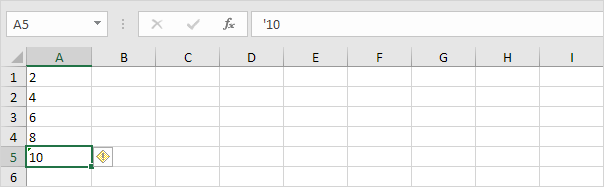3a. If you add text to a number and still want to format this number, use the TEXT function. Without using the TEXT function this would be the result.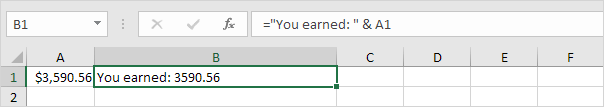3b. With the TEXT function.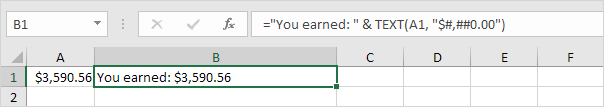Note: #,## is used to add comma's to large numbers.

3c. Here's another example. Apply a percentage format.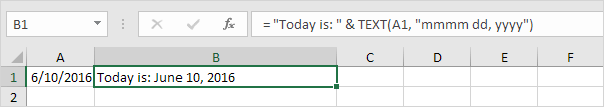Note: use 0 to display the nearest integer value. Use 0.0 for one decimal place. Use 0.00 for two decimal places, etc.

3d. And another example. Format a date.Note: use less/more m's, d's and y's to change the appearance of the date. Visit our page about the TEXT function for many more examples.

#How To#Tutorial#Numbers to Text#Format Cells

Create a custom number format in excel

How to convert text strings that represent numbers to numbers